# RD Sharma Solutions for Class 8 Maths Chapter 13 Profit, Loss, Discount and Value Added Tax (VAT) Exercise 13.2

In order to increase sales or clear stocks sometimes the shopkeeper offers certain percent of rebate on the articles, this rebate is known as the discount. In Exercise 13.2 of Chapter 13, we shall study problems based on discount. To access detailed solutions to problems, students can refer to RD Sharma Solutions which is designed by our expert faculty team at BYJU’S. In order to help students understand the concepts clearly and solve the problems with competence, and also to gain knowledge over the subject, students can use the best shortcut methods explained by our tutors. The pdfs can be easily downloaded from the links provided below.

## Download the Pdf of RD Sharma Solutions for Class 8 Maths Exercise 13.2 Chapter 13 Profit, Loss, Discount and Value Added Tax (VAT)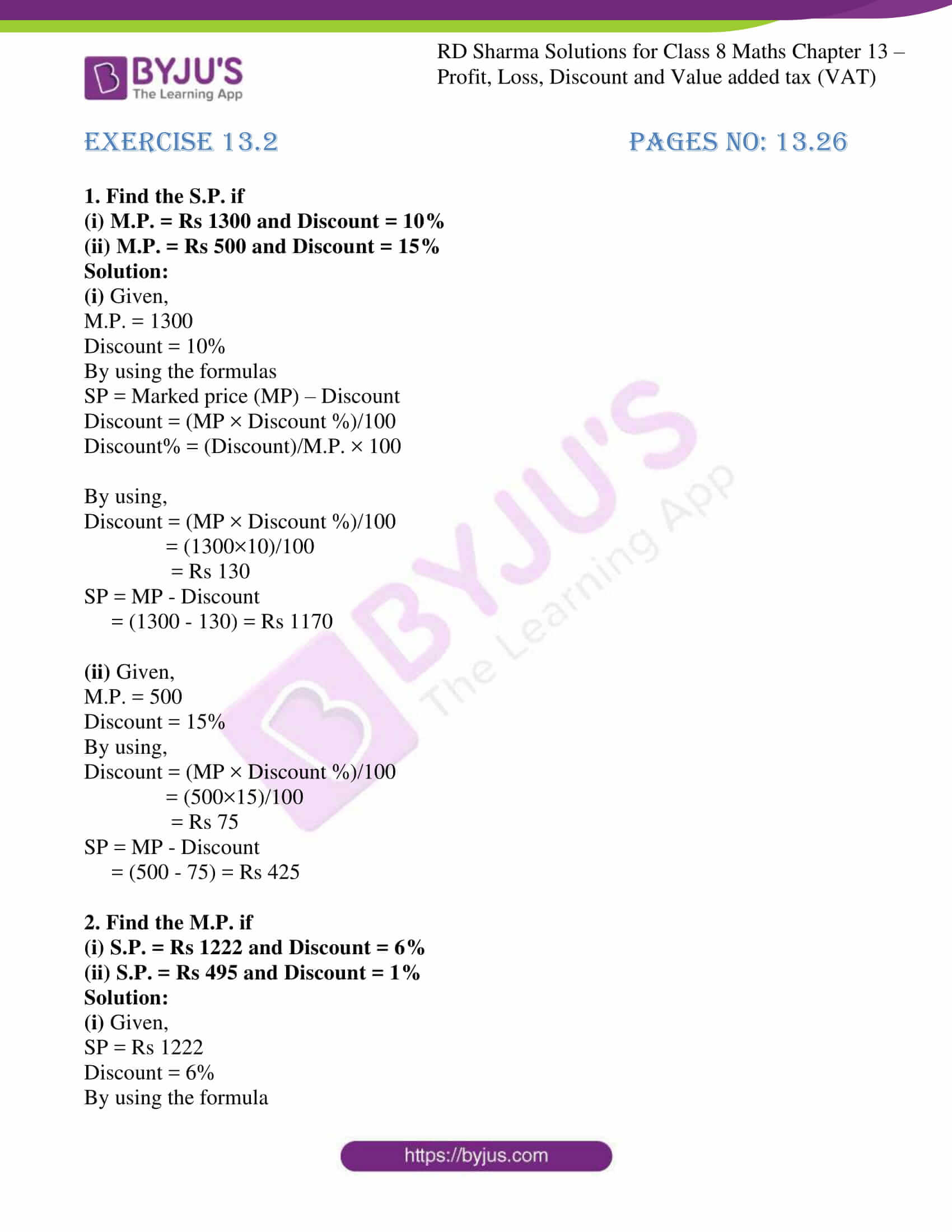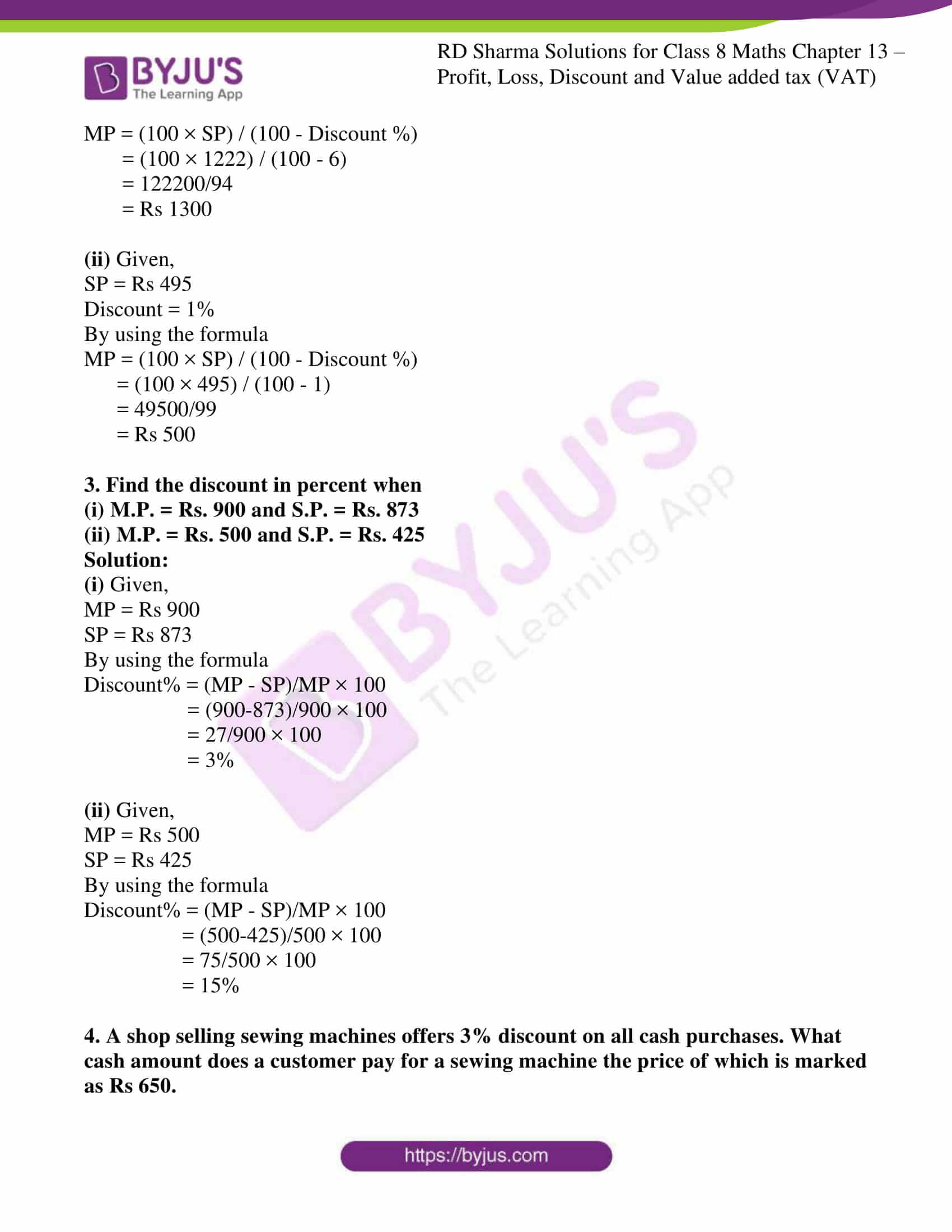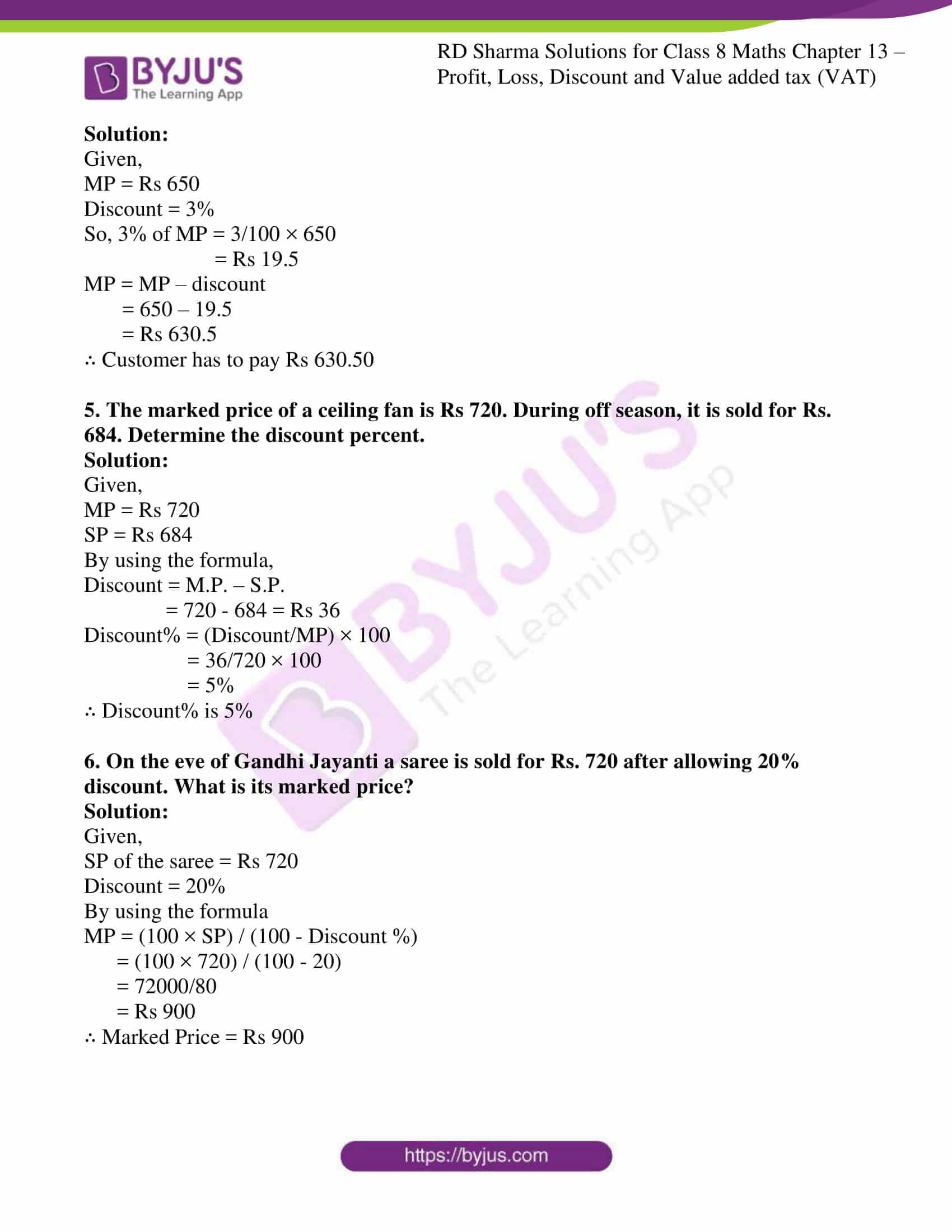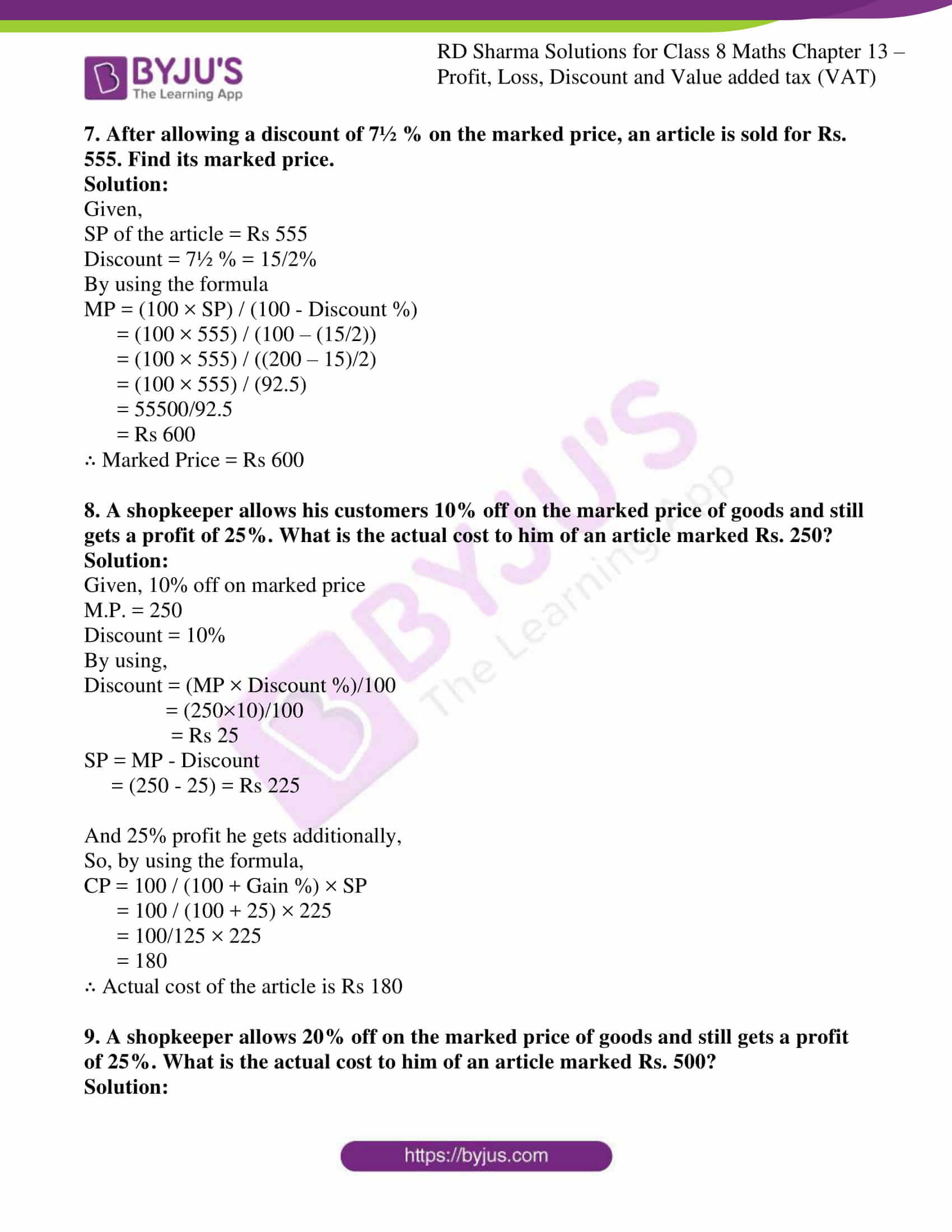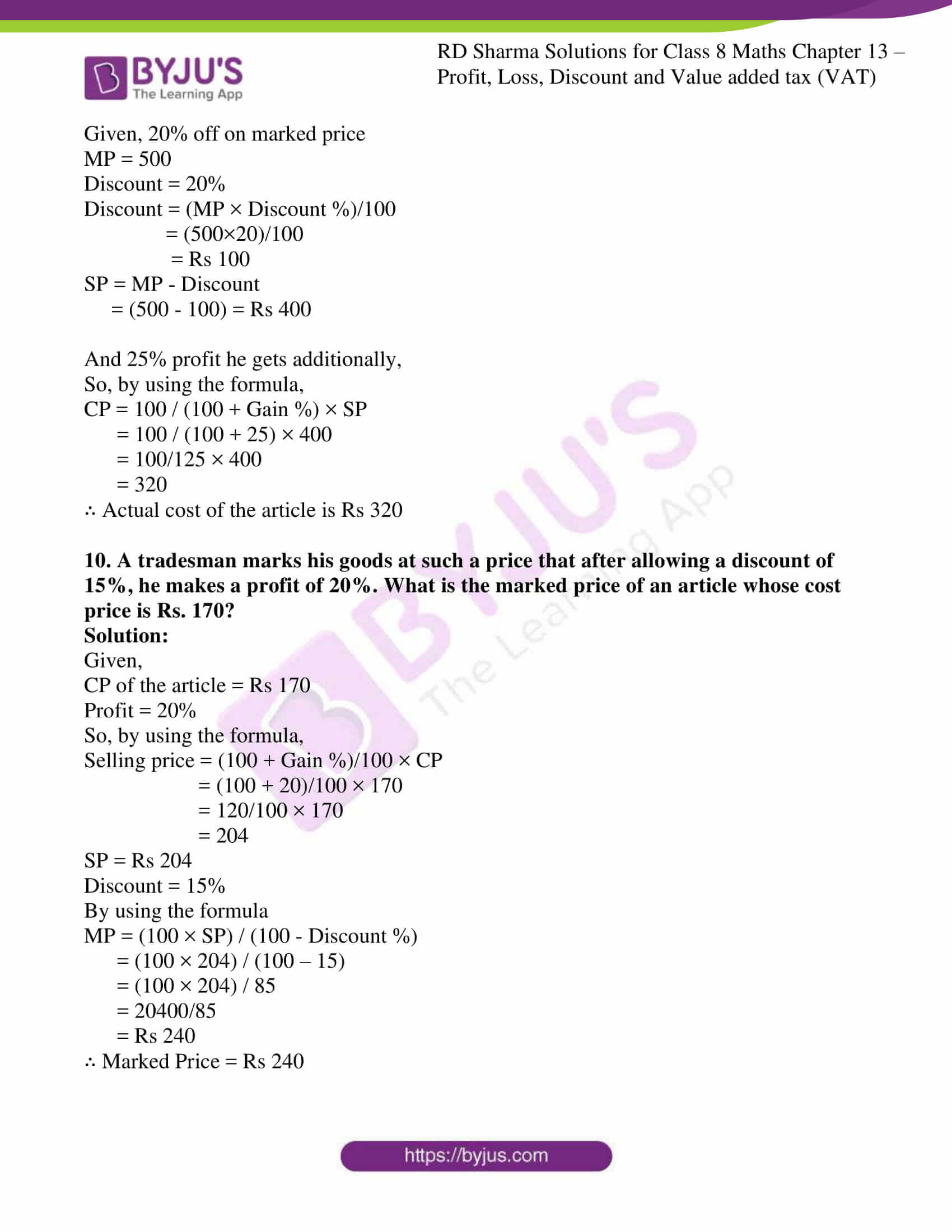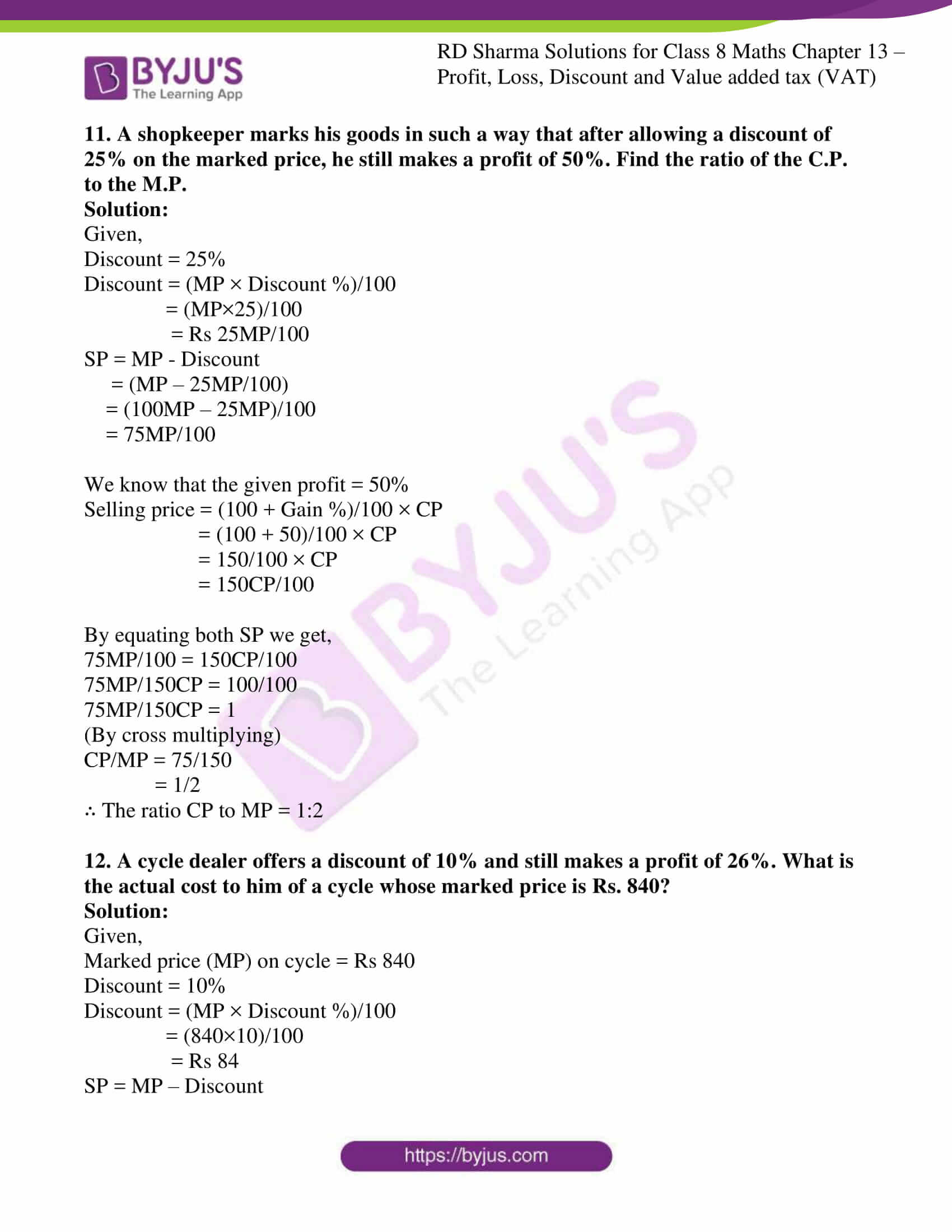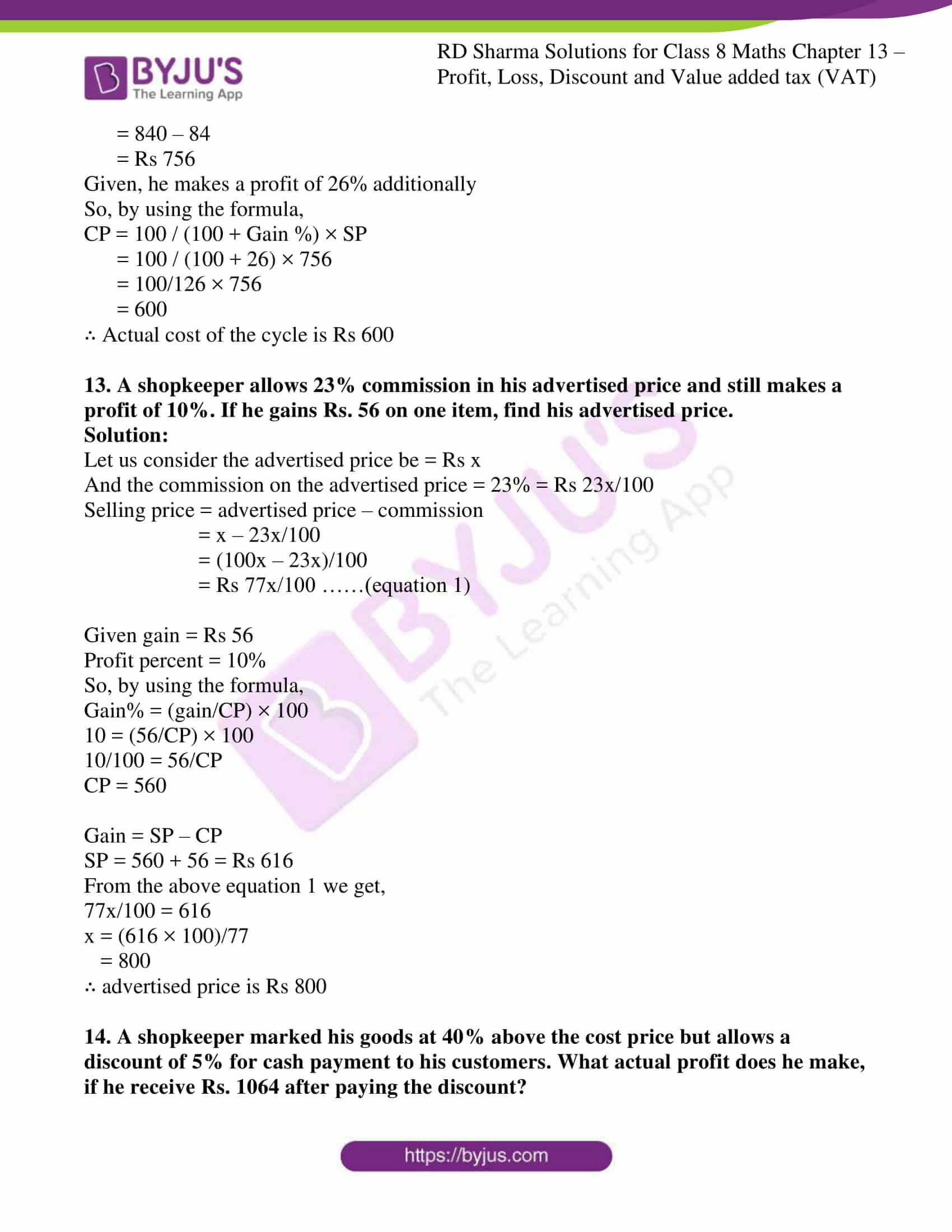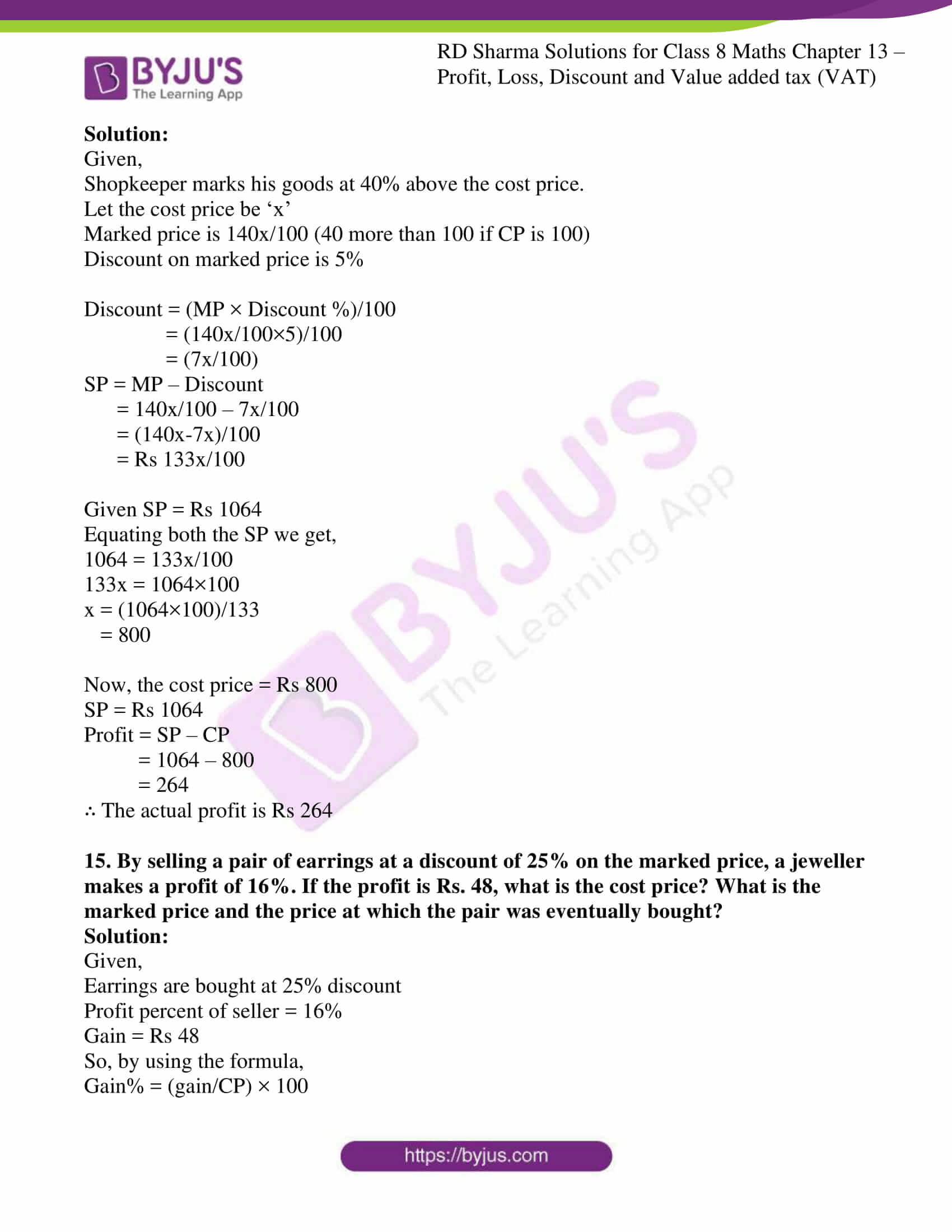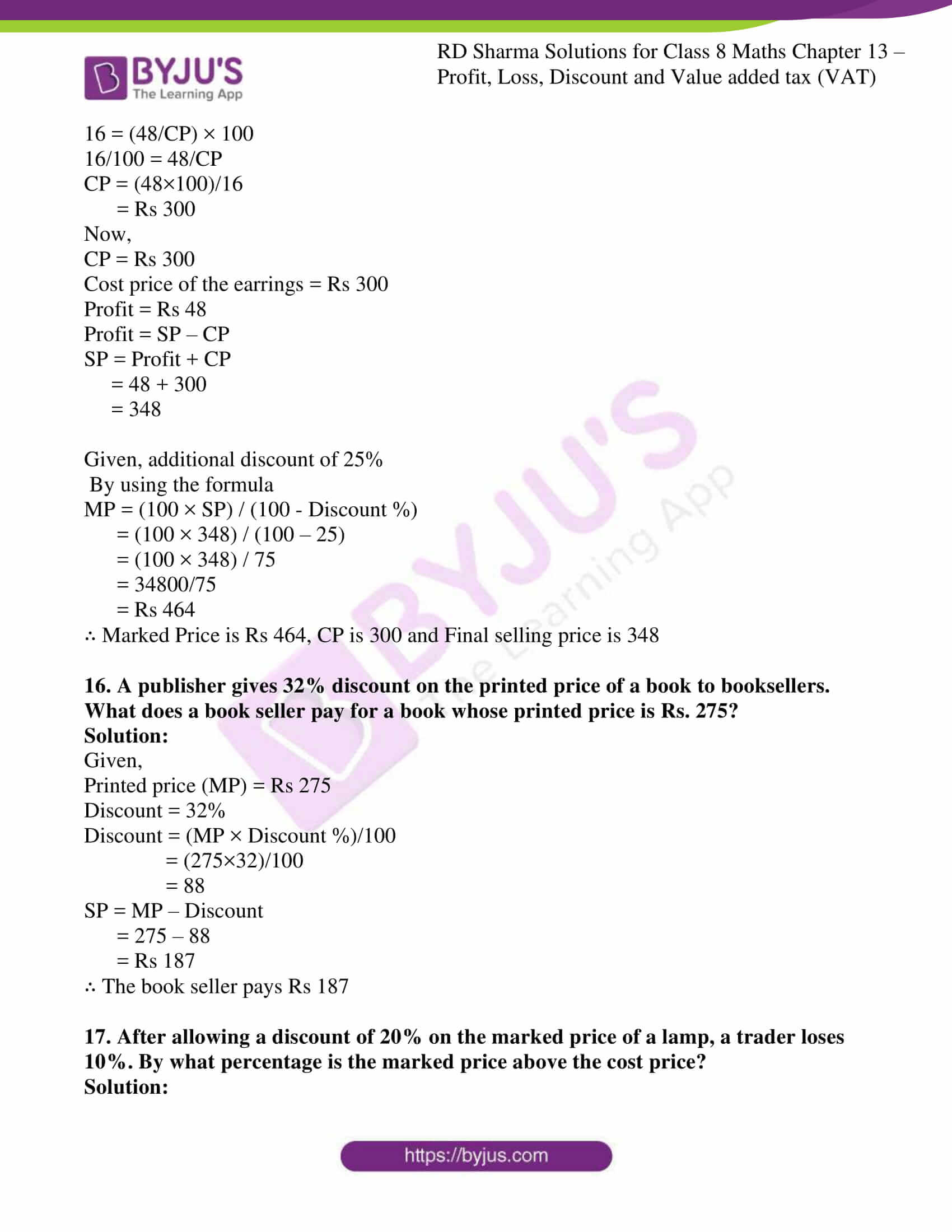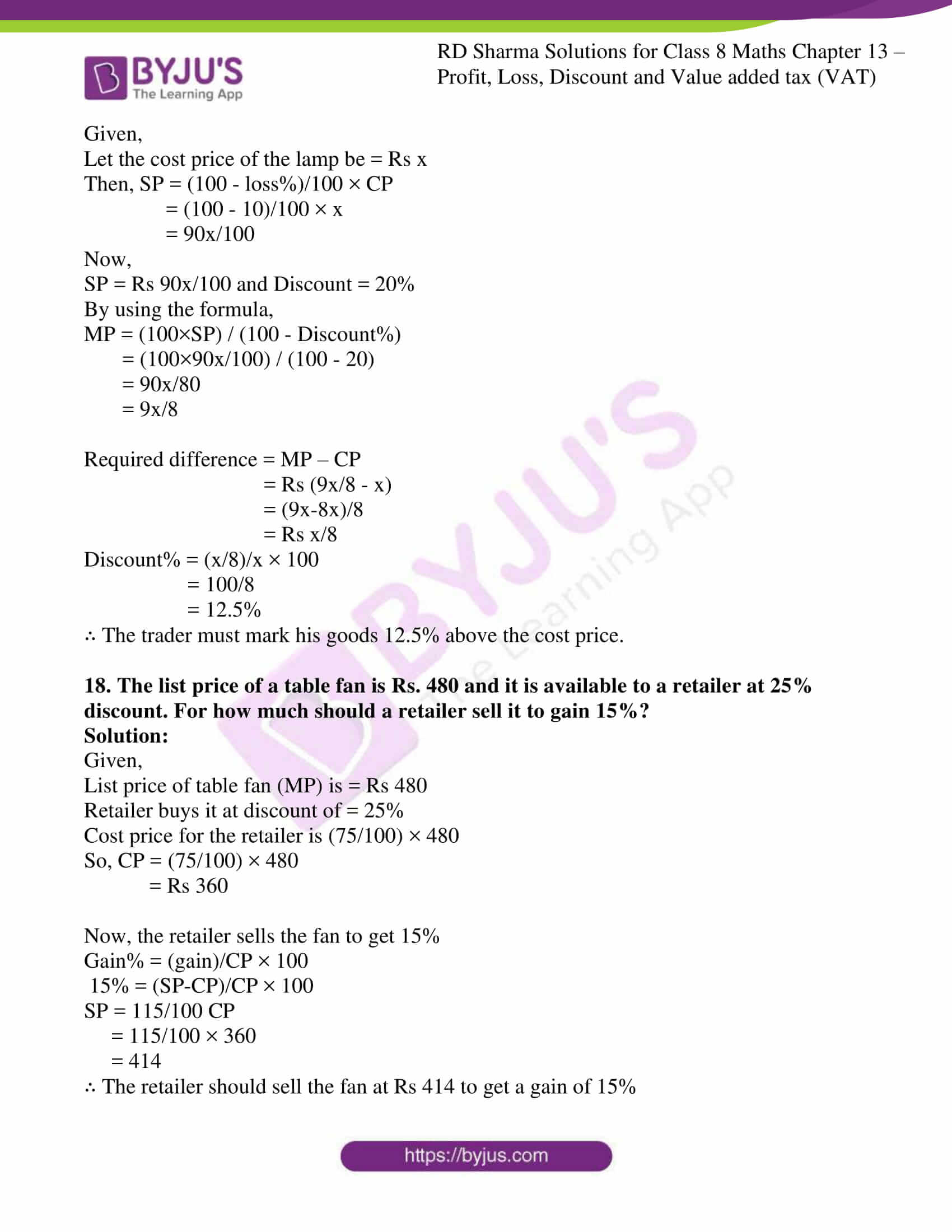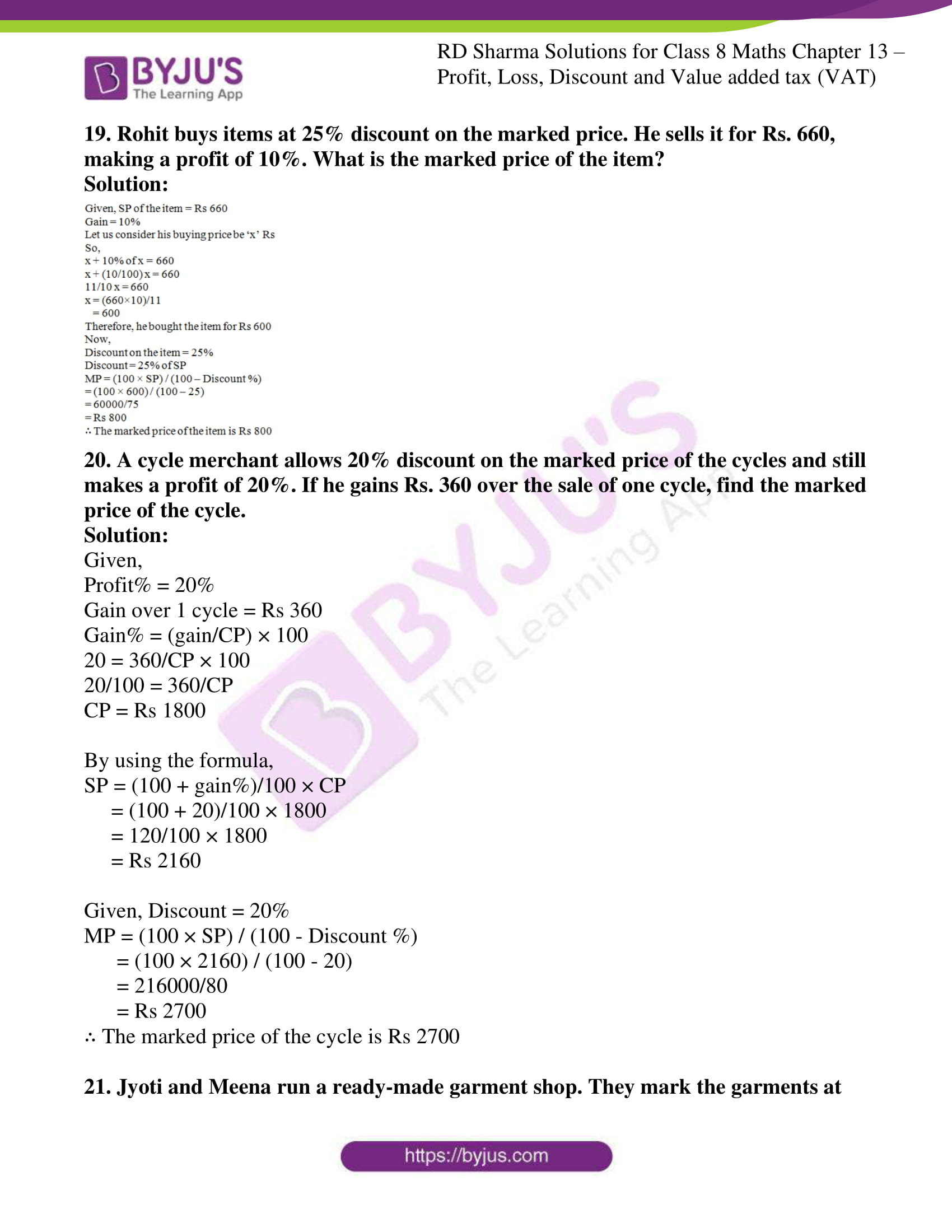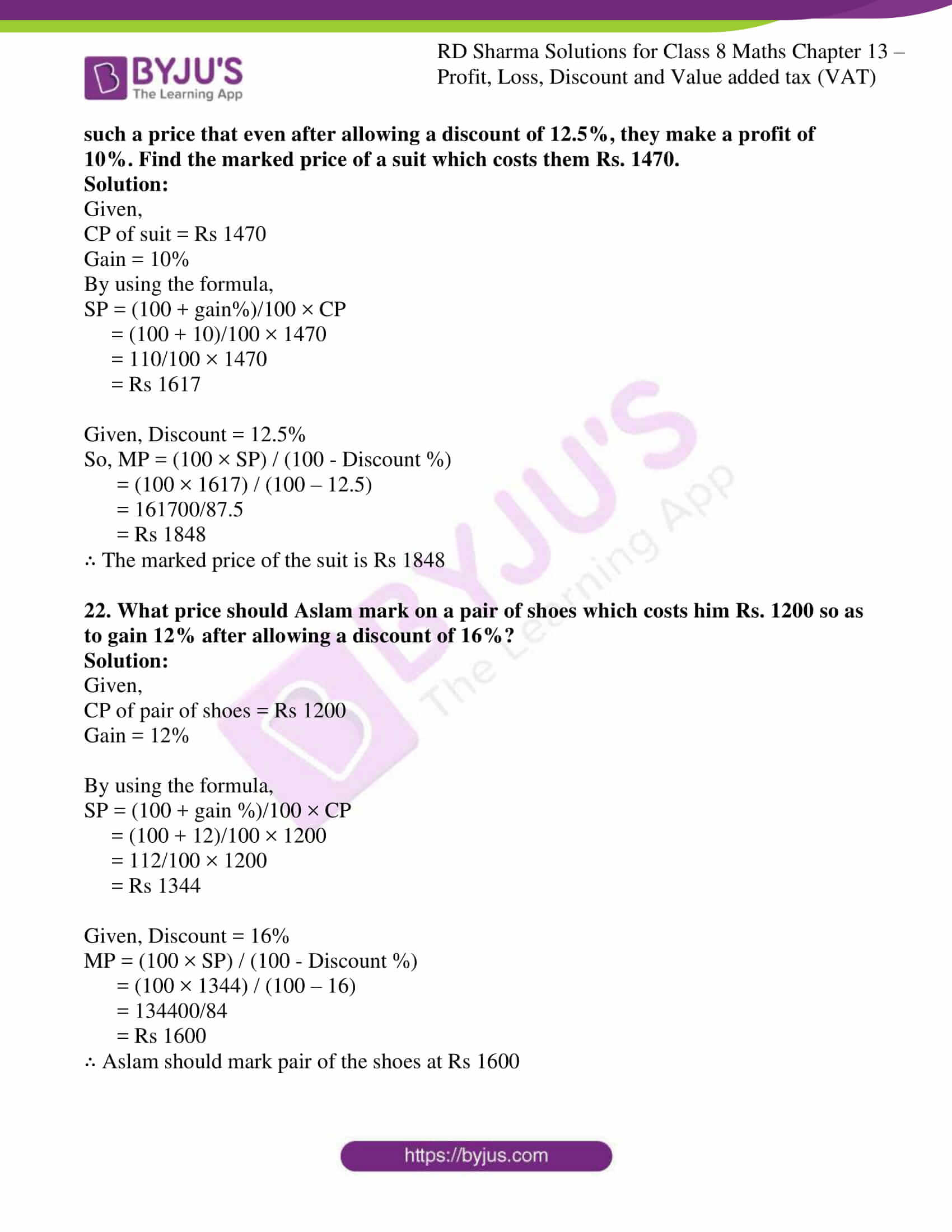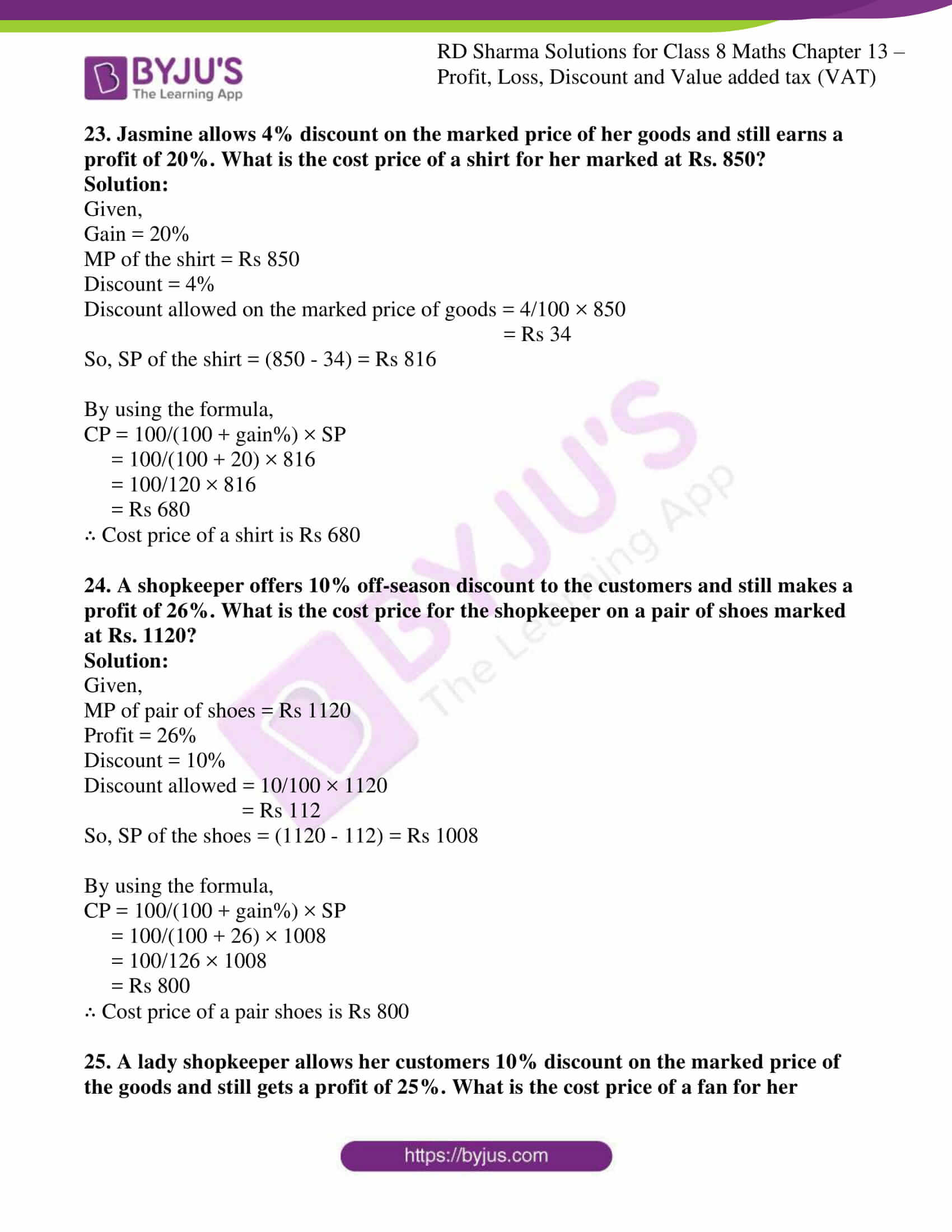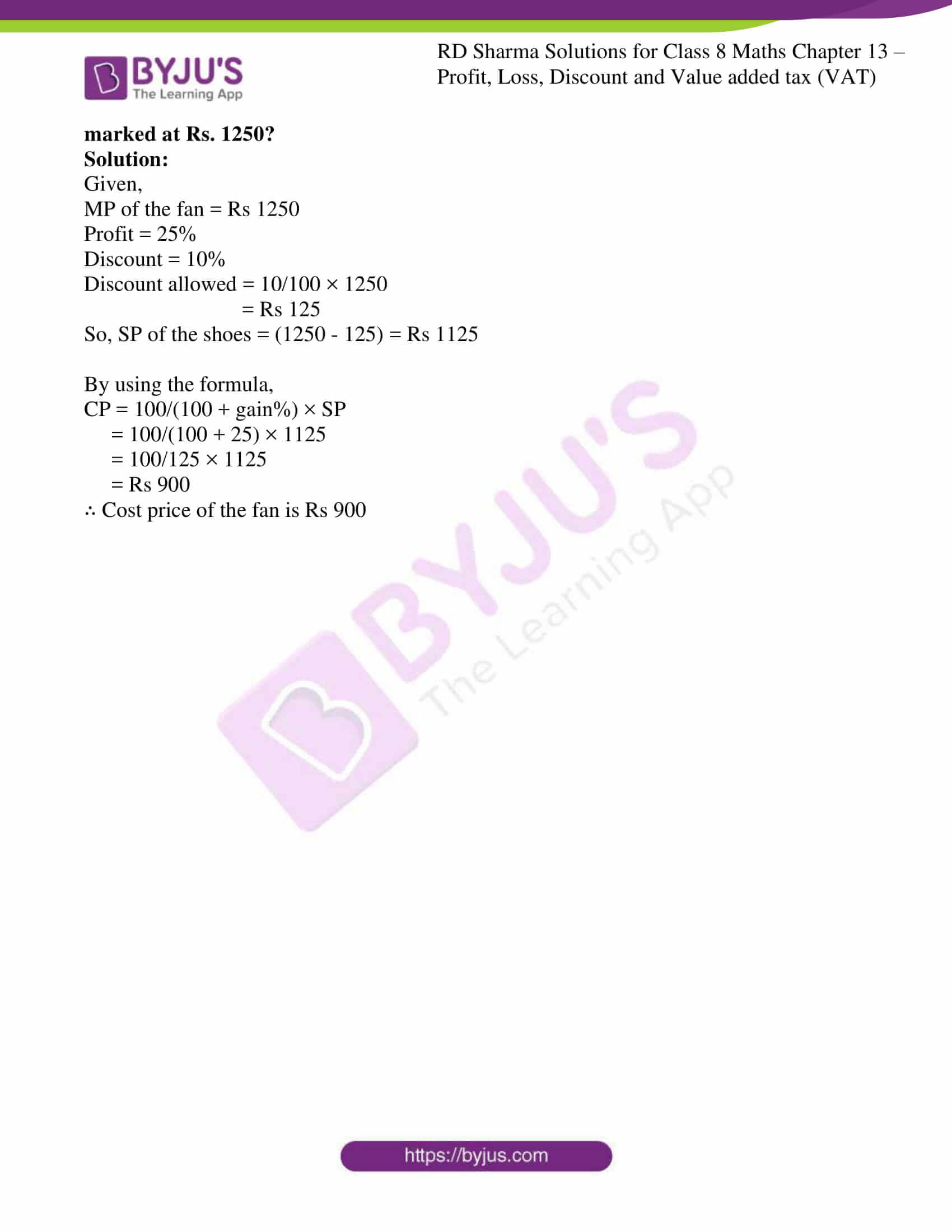### Access answers to Maths RD Sharma Solutions For Class 8 Exercise 13.2 Chapter 13 Profit, Loss, Discount and Value Added Tax (VAT)

1. Find the S.P. if
(i) M.P. = Rs 1300 and Discount = 10%
(ii) M.P. = Rs 500 and Discount = 15%

Solution:

(i) Given,

M.P. = 1300

Discount = 10%

By using the formulas

SP = Marked price (MP) – Discount

Discount = (MP × Discount %)/100

Discount% = (Discount)/M.P. × 100

By using,

Discount = (MP × Discount %)/100

= (1300×10)/100

= Rs 130

SP = MP – Discount

= (1300 – 130) = Rs 1170

(ii) Given,

M.P. = 500

Discount = 15%

By using,

Discount = (MP × Discount %)/100

= (500×15)/100

= Rs 75

SP = MP – Discount

= (500 – 75) = Rs 425

2. Find the M.P. if
(i) S.P. = Rs 1222 and Discount = 6%
(ii) S.P. = Rs 495 and Discount = 1%

Solution:

(i) Given,

SP = Rs 1222

Discount = 6%

By using the formula

MP = (100 × SP) / (100 – Discount %)

= (100 × 1222) / (100 – 6)

= 122200/94

= Rs 1300

(ii) Given,

SP = Rs 495

Discount = 1%

By using the formula

MP = (100 × SP) / (100 – Discount %)

= (100 × 495) / (100 – 1)

= 49500/99

= Rs 500

3. Find the discount in percent when
(i) M.P. = Rs. 900 and S.P. = Rs. 873
(ii) M.P. = Rs. 500 and S.P. = Rs. 425

Solution:

(i) Given,

MP = Rs 900

SP = Rs 873

By using the formula

Discount% = (MP – SP)/MP × 100

= (900-873)/900 × 100

= 27/900 × 100

= 3%

(ii) Given,

MP = Rs 500

SP = Rs 425

By using the formula

Discount% = (MP – SP)/MP × 100

= (500-425)/500 × 100

= 75/500 × 100

= 15%

4. A shop selling sewing machines offers 3% discount on all cash purchases. What cash amount does a customer pay for a sewing machine the price of which is marked as Rs 650.

Solution:

Given,

MP = Rs 650

Discount = 3%

So, 3% of MP = 3/100 × 650

= Rs 19.5

MP = MP – discount

= 650 – 19.5

= Rs 630.5

∴ Customer has to pay Rs 630.50

5. The marked price of a ceiling fan is Rs 720. During off season, it is sold for Rs. 684. Determine the discount percent.

Solution:

Given,

MP = Rs 720

SP = Rs 684

By using the formula,

Discount = M.P. – S.P.

= 720 – 684 = Rs 36

Discount% = (Discount/MP) × 100

= 36/720 × 100

= 5%

∴ Discount% is 5%

6. On the eve of Gandhi Jayanti a saree is sold for Rs. 720 after allowing 20% discount. What is its marked price?

Solution:

Given,

SP of the saree = Rs 720

Discount = 20%

By using the formula

MP = (100 × SP) / (100 – Discount %)

= (100 × 720) / (100 – 20)

= 72000/80

= Rs 900

∴ Marked Price = Rs 900

7. After allowing a discount of 7½ % on the marked price, an article is sold for Rs. 555. Find its marked price.

Solution:

Given,

SP of the article = Rs 555

Discount = 7½ % = 15/2%

By using the formula

MP = (100 × SP) / (100 – Discount %)

= (100 × 555) / (100 – (15/2))

= (100 × 555) / ((200 – 15)/2)

= (100 × 555) / (92.5)

= 55500/92.5

= Rs 600

∴ Marked Price = Rs 600

8. A shopkeeper allows his customers 10% off on the marked price of goods and still gets a profit of 25%. What is the actual cost to him of an article marked Rs. 250?

Solution:

Given, 10% off on marked price

M.P. = 250

Discount = 10%

By using,

Discount = (MP × Discount %)/100

= (250×10)/100

= Rs 25

SP = MP – Discount

= (250 – 25) = Rs 225

And 25% profit he gets additionally,

So, by using the formula,

CP = 100 / (100 + Gain %) × SP

= 100 / (100 + 25) × 225

= 100/125 × 225

= 180

∴ Actual cost of the article is Rs 180

9. A shopkeeper allows 20% off on the marked price of goods and still gets a profit of 25%. What is the actual cost to him of an article marked Rs. 500?

Solution:

Given, 20% off on marked price

MP = 500

Discount = 20%

Discount = (MP × Discount %)/100

= (500×20)/100

= Rs 100

SP = MP – Discount

= (500 – 100) = Rs 400

And 25% profit he gets additionally,

So, by using the formula,

CP = 100 / (100 + Gain %) × SP

= 100 / (100 + 25) × 400

= 100/125 × 400

= 320

∴ Actual cost of the article is Rs 320

10. A tradesman marks his goods at such a price that after allowing a discount of 15%, he makes a profit of 20%. What is the marked price of an article whose cost price is Rs. 170?

Solution:

Given,

CP of the article = Rs 170

Profit = 20%

So, by using the formula,

Selling price = (100 + Gain %)/100 × CP

= (100 + 20)/100 × 170

= 120/100 × 170

= 204

SP = Rs 204

Discount = 15%

By using the formula

MP = (100 × SP) / (100 – Discount %)

= (100 × 204) / (100 – 15)

= (100 × 204) / 85

= 20400/85

= Rs 240

∴ Marked Price = Rs 240

11. A shopkeeper marks his goods in such a way that after allowing a discount of 25% on the marked price, he still makes a profit of 50%. Find the ratio of the C.P. to the M.P.

Solution:

Given,

Discount = 25%

Discount = (MP × Discount %)/100

= (MP×25)/100

= Rs 25MP/100

SP = MP – Discount

= (MP – 25MP/100)

= (100MP – 25MP)/100

= 75MP/100

We know that the given profit = 50%

Selling price = (100 + Gain %)/100 × CP

= (100 + 50)/100 × CP

= 150/100 × CP

= 150CP/100

By equating both SP we get,

75MP/100 = 150CP/100

75MP/150CP = 100/100

75MP/150CP = 1

(By cross multiplying)

CP/MP = 75/150

= 1/2

∴ The ratio CP to MP = 1:2

12. A cycle dealer offers a discount of 10% and still makes a profit of 26%. What is the actual cost to him of a cycle whose marked price is Rs. 840?

Solution:

Given,

Marked price (MP) on cycle = Rs 840

Discount = 10%

Discount = (MP × Discount %)/100

= (840×10)/100

= Rs 84

SP = MP – Discount

= 840 – 84

= Rs 756

Given, he makes a profit of 26% additionally

So, by using the formula,

CP = 100 / (100 + Gain %) × SP

= 100 / (100 + 26) × 756

= 100/126 × 756

= 600

∴ Actual cost of the cycle is Rs 600

13. A shopkeeper allows 23% commission in his advertised price and still makes a profit of 10%. If he gains Rs. 56 on one item, find his advertised price.

Solution:

Let us consider the advertised price be = Rs x

And the commission on the advertised price = 23% = Rs 23x/100

Selling price = advertised price – commission

= x – 23x/100

= (100x – 23x)/100

= Rs 77x/100 ……(equation 1)

Given gain = Rs 56

Profit percent = 10%

So, by using the formula,

Gain% = (gain/CP) × 100

10 = (56/CP) × 100

10/100 = 56/CP

CP = 560

Gain = SP – CP

SP = 560 + 56 = Rs 616

From the above equation 1 we get,

77x/100 = 616

x = (616 × 100)/77

= 800

∴ advertised price is Rs 800

14. A shopkeeper marked his goods at 40% above the cost price but allows a discount of 5% for cash payment to his customers. What actual profit does he make, if he receive Rs. 1064 after paying the discount?

Solution:

Given,

Shopkeeper marks his goods at 40% above the cost price.

Let the cost price be ‘x’

Marked price is 140x/100 (40 more than 100 if CP is 100)

Discount on marked price is 5%

Discount = (MP × Discount %)/100

= (140x/100×5)/100

= (7x/100)

SP = MP – Discount

= 140x/100 – 7x/100

= (140x-7x)/100

= Rs 133x/100

Given SP = Rs 1064

Equating both the SP we get,

1064 = 133x/100

133x = 1064×100

x = (1064×100)/133

= 800

Now, the cost price = Rs 800

SP = Rs 1064

Profit = SP – CP

= 1064 – 800

= 264

∴ The actual profit is Rs 264

15. By selling a pair of earrings at a discount of 25% on the marked price, a jeweller makes a profit of 16%. If the profit is Rs. 48, what is the cost price? What is the marked price and the price at which the pair was eventually bought?

Solution:

Given,

Earrings are bought at 25% discount

Profit percent of seller = 16%

Gain = Rs 48

So, by using the formula,

Gain% = (gain/CP) × 100

16 = (48/CP) × 100

16/100 = 48/CP

CP = (48×100)/16

= Rs 300

Now,

CP = Rs 300

Cost price of the earrings = Rs 300

Profit = Rs 48

Profit = SP – CP

SP = Profit + CP

= 48 + 300

= 348

By using the formula

MP = (100 × SP) / (100 – Discount %)

= (100 × 348) / (100 – 25)

= (100 × 348) / 75

= 34800/75

= Rs 464

∴ Marked Price is Rs 464, CP is 300 and Final selling price is 348

16. A publisher gives 32% discount on the printed price of a book to booksellers. What does a book seller pay for a book whose printed price is Rs. 275?

Solution:

Given,

Printed price (MP) = Rs 275

Discount = 32%

Discount = (MP × Discount %)/100

= (275×32)/100

= 88

SP = MP – Discount

= 275 – 88

= Rs 187

∴ The book seller pays Rs 187

17. After allowing a discount of 20% on the marked price of a lamp, a trader loses 10%. By what percentage is the marked price above the cost price?

Solution:

Given,

Let the cost price of the lamp be = Rs x

Then, SP = (100 – loss%)/100 × CP

= (100 – 10)/100 × x

= 90x/100

Now,

SP = Rs 90x/100 and Discount = 20%

By using the formula,

MP = (100×SP) / (100 – Discount%)

= (100×90x/100) / (100 – 20)

= 90x/80

= 9x/8

Required difference = MP – CP

= Rs (9x/8 – x)

= (9x-8x)/8

= Rs x/8

Discount% = (x/8)/x × 100

= 100/8

= 12.5%

∴ The trader must mark his goods 12.5% above the cost price.

18. The list price of a table fan is Rs. 480 and it is available to a retailer at 25% discount. For how much should a retailer sell it to gain 15%?

Solution:

Given,

List price of table fan (MP) is = Rs 480

Retailer buys it at discount of = 25%

Cost price for the retailer is (75/100) × 480

So, CP = (75/100) × 480

= Rs 360

Now, the retailer sells the fan to get 15%

Gain% = (gain)/CP × 100

15% = (SP-CP)/CP × 100

SP = 115/100 CP

= 115/100 × 360

= 414

∴ The retailer should sell the fan at Rs 414 to get a gain of 15%

19. Rohit buys items at 25% discount on the marked price. He sells it for Rs. 660, making a profit of 10%. What is the marked price of the item?

Solution: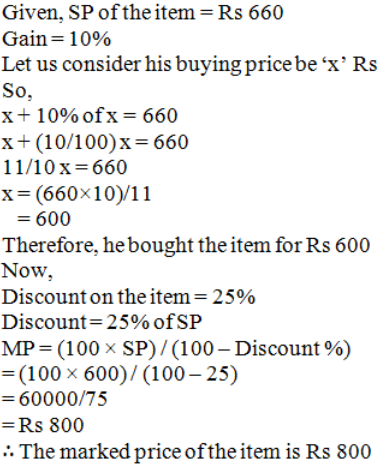20. A cycle merchant allows 20% discount on the marked price of the cycles and still makes a profit of 20%. If he gains Rs. 360 over the sale of one cycle, find the marked price of the cycle.

Solution:

Given,

Profit% = 20%

Gain over 1 cycle = Rs 360

Gain% = (gain/CP) × 100

20 = 360/CP × 100

20/100 = 360/CP

CP = Rs 1800

By using the formula,

SP = (100 + gain%)/100 × CP

= (100 + 20)/100 × 1800

= 120/100 × 1800

= Rs 2160

Given, Discount = 20%

MP = (100 × SP) / (100 – Discount %)

= (100 × 2160) / (100 – 20)

= 216000/80

= Rs 2700

∴ The marked price of the cycle is Rs 2700

21. Jyoti and Meena run a ready-made garment shop. They mark the garments at such a price that even after allowing a discount of 12.5%, they make a profit of 10%. Find the marked price of a suit which costs them Rs. 1470.

Solution:

Given,

CP of suit = Rs 1470

Gain = 10%

By using the formula,

SP = (100 + gain%)/100 × CP

= (100 + 10)/100 × 1470

= 110/100 × 1470

= Rs 1617

Given, Discount = 12.5%

So, MP = (100 × SP) / (100 – Discount %)

= (100 × 1617) / (100 – 12.5)

= 161700/87.5

= Rs 1848

∴ The marked price of the suit is Rs 1848

22. What price should Aslam mark on a pair of shoes which costs him Rs. 1200 so as to gain 12% after allowing a discount of 16%?

Solution:

Given,

CP of pair of shoes = Rs 1200

Gain = 12%

By using the formula,

SP = (100 + gain %)/100 × CP

= (100 + 12)/100 × 1200

= 112/100 × 1200

= Rs 1344

Given, Discount = 16%

MP = (100 × SP) / (100 – Discount %)

= (100 × 1344) / (100 – 16)

= 134400/84

= Rs 1600

∴ Aslam should mark pair of the shoes at Rs 1600

23. Jasmine allows 4% discount on the marked price of her goods and still earns a profit of 20%. What is the cost price of a shirt for her marked at Rs. 850?

Solution:

Given,

Gain = 20%

MP of the shirt = Rs 850

Discount = 4%

Discount allowed on the marked price of goods = 4/100 × 850

= Rs 34

So, SP of the shirt = (850 – 34) = Rs 816

By using the formula,

CP = 100/(100 + gain%) × SP

= 100/(100 + 20) × 816

= 100/120 × 816

= Rs 680

∴ Cost price of a shirt is Rs 680

24. A shopkeeper offers 10% off-season discount to the customers and still makes a profit of 26%. What is the cost price for the shopkeeper on a pair of shoes marked at Rs. 1120?

Solution:

Given,

MP of pair of shoes = Rs 1120

Profit = 26%

Discount = 10%

Discount allowed = 10/100 × 1120

= Rs 112

So, SP of the shoes = (1120 – 112) = Rs 1008

By using the formula,

CP = 100/(100 + gain%) × SP

= 100/(100 + 26) × 1008

= 100/126 × 1008

= Rs 800

∴ Cost price of a pair shoes is Rs 800

25. A lady shopkeeper allows her customers 10% discount on the marked price of the goods and still gets a profit of 25%. What is the cost price of a fan for her marked at Rs. 1250?

Solution:

Given,

MP of the fan = Rs 1250

Profit = 25%

Discount = 10%

Discount allowed = 10/100 × 1250

= Rs 125

So, SP of the shoes = (1250 – 125) = Rs 1125

By using the formula,

CP = 100/(100 + gain%) × SP

= 100/(100 + 25) × 1125

= 100/125 × 1125

= Rs 900

∴ Cost price of the fan is Rs 900